Courses

# Test: Previous Year Questions - Electrochemistry (Level 1)

## 21 Questions MCQ Test Chemistry Class 12 | Test: Previous Year Questions - Electrochemistry (Level 1)

Description
This mock test of Test: Previous Year Questions - Electrochemistry (Level 1) for Class 12 helps you for every Class 12 entrance exam. This contains 21 Multiple Choice Questions for Class 12 Test: Previous Year Questions - Electrochemistry (Level 1) (mcq) to study with solutions a complete question bank. The solved questions answers in this Test: Previous Year Questions - Electrochemistry (Level 1) quiz give you a good mix of easy questions and tough questions. Class 12 students definitely take this Test: Previous Year Questions - Electrochemistry (Level 1) exercise for a better result in the exam. You can find other Test: Previous Year Questions - Electrochemistry (Level 1) extra questions, long questions & short questions for Class 12 on EduRev as well by searching above.
QUESTION: 1

Solution:
QUESTION: 2

### Which of the following reactions is possible at anode ?            [AIEEE-2002]

Solution:

The correct answer is Option C.

The anode is the electrode where oxidation (loss of electrons) takes place; in a galvanic cell, it is the negative electrode, as when oxidation occurs, electrons are left behind on the electrode.

The cathode is the electrode where reduction (gain of electrons) takes place; in a galvanic cell, it is the positive electrode, as less oxidation occurs, fewer ions go into solution, and fewer electrons are left on the electrode.
So, in the reaction
2Cr3+ +7H2O→Cr2O72−+14H+

Cr is getting oxidised as the oxidation state is changing from +3 to +6 and hence this reaction is possible at anode.

*Multiple options can be correct
QUESTION: 3

### For a cell given below Ag | Ag+ || Cu2+ | Cu  —              +  Ag+ + e- → Ag,      Eº = x  Cu2+ +2e- → Cu,    Eº = y  Eº cell is –                     [AIEEE-2002]

Solution:

The correct answer is Option C.

The left portion of the salt bridge(| |)  is anode and the right portion of the salt bridge(| |) is cathode.

Since, Ag | Ag+ is half-cell oxidation and Cu2+ | Cu is half-cell reduction. Thus,
Eo cell = E cathode - E anode
= y - x

*Multiple options can be correct
QUESTION: 4

For a cell reaction involving a two-electron change the standard e.m.f. of the cell is found to be 0.295 V at 25ºC. The equilibrium constant of the reaction at 25º C will be –

[AIEEE-2003]

Solution:

The correct answer is Option B.
Eocell = (0.0591/n) log Kc
0.259 = (0.0591/2) log Kc
0.259 = 0.0295 log Kc
Kc = antilog 10
Kc = 1 x 1010

*Multiple options can be correct
QUESTION: 5

Standard reduction electrode potentials of three metals A, B and C are respectively +0.5 V, – 3.0V and – 1.2 V. The reducing powers of these metals are -

[AIEEE-2003]

Solution:

The correct answer is option C
A       B        C
red +0.5V –3.0V –1.2V
More is the value of reduction potential, more is the tendency to get reduced, i.e. less is the reducing power. The reducing power follows the following order: B > C > A.

*Multiple options can be correct
QUESTION: 6

For the redox reaction :

Zn (s) + Cu2+ (0.1M) → Zn2+ (1M) + Cu(s) taking place in a cell, Eºcell is 1.10 volt. Ecell for the cell will be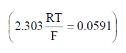[AIEEE-2003]

Solution:

The correct answer is Option A.
Eocell =  Ecell – (0.0591/n) log (Zn2+/ Cu2+)
= 1.10 – (0.0591/n) log (1 / 0.1)
= 1.10 – 0.02955
= 1.070 Volt

QUESTION: 7

When during electrolysis of a solution of AgNO3 9650 coulombs of charge pass through the electroplating bath, the mass of silver deposited on the cathode will be –

[AIEEE-2003]

Solution:

The correct answer is Option D.
Ag+ + e- ---> Ag
So, no. of the moles of Ag = Q / F
= 9650 / 96500
=1 / 10 moles
∴ Mass of Ag produced :->
(1/10) × 108
= 10.8 g

*Multiple options can be correct
QUESTION: 8

Consider the following E° values

[AIEEE-2004]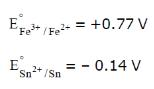under standard conditions the potential for the reaction

Sn(s) + 2Fe3+ (aq) → 2Fe2+ (aq) + Sn2+ (aq) is

Solution:

The correct answer is option C
E0cell ​= Eooxd ​+ Eored​
= Eo Sn/Sn2+ ​+ EoFe3+/Fe2+​
Given : Eo​Sn2+/Sn = −0.14 ⇒EoSn/Sn2+​=−(−0.14)
=0.14V
∴ Eocell ​= 0.14 + 0.77 = 0.91V

QUESTION: 9

The standard e.m.f. of a cell, involving one electron change is found to be 0.591 V at 25°C. The equilibrium constant of the reaction is (F = 96500 C mol-1 ; R = 8.314 JK-1mol-1)

[AIEEE-2004]

Solution:

The correct answer is option C
For a cell reaction in equilibrium at 298 K,
Eocell =0.0591/nlogKc
(Ke = equilibrium constant)
Give,     Eocell = 0.591V
Now, log Kc = (Eocell x n )/ 0.0591
=(0.0591 x n )/ 0.0591
log Kc = 10
Kc = antilog 10

1. Kc = 1 x 1010
QUESTION: 10

The limiting molar conductivities ^° for NaCl, KBr and KCl are 126, 152 and 150 S cm2 mol-1 respectively. The ^° for NaBr is –

[AIEEE-2004]

Solution:

The correct answer is Option A.

The limiting molar conductivities of NaCl, KBr and KCl are 126, 152 and 150 Scm2mol−1 respectively.
The limiting molar conductivity  Λo for NaBr is calculated by the following expression.
λNaBr = λNaCl + λKBr + λKCl
λNaBr = 126+152−150=128 Scm.2mol−1

QUESTION: 11

In a cell that utilises the reaction

Zn(s) + 2H+ (aq)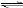Zn2+ (aq) + H2(g)

addition of H2SO4 to cathode compartment, will–

[AIEEE-2004]

Solution:

The correct answer is Option C.

Zn(s) +2H+ (aq) →Zn2+(aq)+H2(g)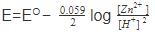So as we add sulphuric acid, conc. of hydrogen ion increases and equilibrium shifts to right.
Also according to the equation emf of the cell will be increased by adding acid.

QUESTION: 12

Aluminium oxide may be electrolysed at 1000ºC to furnish aluminium metal (At. Mass=27 amu ; 1 Faraday = 96,500 Coulombs). The cathode reaction is

Al3+ + 3e-→ Alº

To prepare 5.12 kg of aluminium metal by this method would require -

[AIEEE-2005]

Solution:

The correct answer is option B
Al3+ + 3e− → Al
w = zQ
where w = amount of metal
=5.12kg = 5.12 × 103g
z = electrochemical equivalent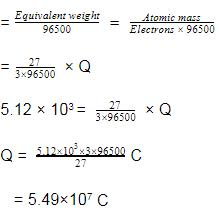QUESTION: 13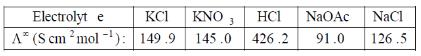Calculate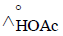using appropriate molar conductance of the electrolytes listed above at infinite dilution in H2O at 25ºC

[AIEEE-2005]

Solution:

The correct answer is option D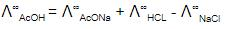= 91.0 +426.2 - 126.5
= 390.7 S cm2 mol-1 .

QUESTION: 14

For a spontaneous reaction the ΔG, equilibrium constant (K) and Ecell will be respectively -

[AIEEE-2005]

Solution:

The correct answer is option B
we know  ΔG=−RTlnK=−nFE∘
We know for a spontaneous reaction
ΔG<0.
∴−RTlnK<0
∴lnK>0
∴K>1
and  −nFE∘<0
∴Ecell∘​>0

QUESTION: 15

Given the data at 25ºC,

Ag + I- → AgI + e-                     Eº = 0.152 V

Ag → Ag+ + e-                          Eº = -0.800 V

What is the value of log Ksp for AgI ?

(2.303 RT/F = 0.059 V)

[AIEEE 2006]

Solution:

The correct answer is option C
AgI (s) + e ⇌ Ag(s) + I;
E∘ = −0.152 + Ag(s) → Ag+ + e
E∘ = −0.8
Agl (s) → Ag+ + I
E∘ = −0.952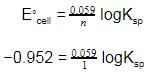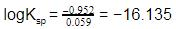QUESTION: 16

The cell, Zn | Zn2+ (1M) | | Cu2+(1M)|Cu (Eºcell)=1.10 V), was allowed to be completely discharged at 298 K.

the relative concentration of zn2+ to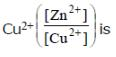Solution:

The correct answer is option C
If it is allowed to completely discharge, Ecell​=0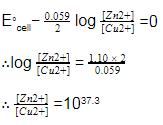QUESTION: 17

The equivalent conductance of two strong electrolytes at infinite dilution in H2O (where ions move freely through a solution) at 25ºC are given below -

^°CH3COONa= 91.0 S cm2/equiv

HCl = 426.2 S cm2/equiv

What additional information/quantity one needs to calculate Lº of an aqueous solution of acetic acid ?

[AIEEE 2007]

Solution:
QUESTION: 18

Given EºCr3+/Cr = – 0.72 V, EºFe2+/Fe= – 0.42 V. The potential for the cell Cr |Cr3+ (0.1 M)| |Fe2+ (0.01 M) | Fe is -

[AIEEE 2008]

Solution:

The correct answer is option D
Cr∣Cr3+(0.1 M)∣∣Fe2+(0.01 M)∣Fe
Oxidation half-cell
Cr→Cr3++3e]×2
Reduction half-cell
Fe2++2e−→Fe]×3
Net cell reaction
2Cr+3Fe2+→2Cr3++3Fe, n=6
E∘​cell​= Eoxi​+E​red
=0.72−0.42 = 0.30 V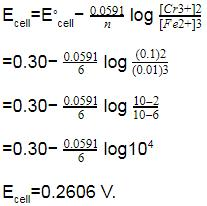QUESTION: 19

The standard reduction potentials for Zn2+/Zn, Ni2+ /Ni, and Fe2+/Fe are – 0.76, – 0.23 and – 0.44 V respectively. The reaction X + Y2+ → X2+ + Y will be spontaneous when –

[AIEEE 2012]

Solution:

The correct answer is option C
The elements with high negative value of standard reduction potential are good reducing agents and can be easily oxidised. ltbRgt Thus X should have a high negative value of standard potential then Y, so that it will be oxidised to X2+ by reducing Y2+ to Y.
X= Zn, Y= Ni
Zn + Ni2+ → Zn2+ + Ni
Alternatively, for a spontaneous reaction E∘ must be positive.
E∘=E∘reduced−E∘oxidised
=−0.23−(0.76)
implies E∘=+0.53V

QUESTION: 20

Refining of impure copper with zinc impurity is to be done by electrolysis using electrods as –

[AIEEE-2002]

Solution:

Cathode is always taken of pure substance because cathode is for reduction. at anode oxidation takes place and the metal oxidises itself into cation and moves to cathode, and gets deposited there. Whereas the impurities settle down below anode as anode mud.

QUESTION: 21

Given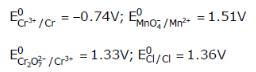Based on the data given above, strongest oxidisting agent will be

[AIEEE 2013]

Solution:

Higher the SRP, better is oxidizing agent hence
MnO−4
MnO4- is the strongest oxidizing agent.